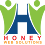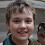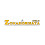# Support my work on Patreon

If you like my work, please consider to help my growing family with a little financial support through my Patreon creator page.

Alternatively, you can leave a one-time donation via PayPal using the Donate button below.

----------------

## Introduction

This post describes the new shadows/highlights adjustment tool that I have introduced in PhotoFlow, and gives some implementation details in case someone would like to port the code to some other image editor.

1. the input pixels are converted from linear RGB to log grayscale, to create a log-luminance (LL) mask:
LL = log10(lumi(RGB))
2. the LL mask is blurred with a guided filter pyramid (more on this below)
3. the blurred LL mask is tone-mapped with an analytical function that rises the shadows and lowers the highlights (more on this below); an anchor parameter allows to define the splitting point between shadows and highlights regions
4. the input RGB values are linearly scaled by the ratio of the blurred LL mask after/before the tone mapping

The tone compression function, applied to the input image without any blurring, results in flattened mid-tones and in a loss of local contrast. The solution is to apply the tone-mapping to a blurred version of the input image, so that local contrast is preserved in the process. However, simple blur filters like gaussian result in visible and disturbing halos around sharp edges. The solution is to use edge-preserving blur filters, which are usually controlled by a threshold parameter that separates sharp edges (which should be preserved) from textures (which should be blurred).

Among the various edge-preserving blur filters, the guided filter is of particular interest for our purpose, because it avoids the over-sharpening artefacts sometimes found in other filters like the bilateral blur. However, in order to obtain an optimal output from the guided filter, some tricks are needed. Let me illustrate them using this test image, with dark and bright areas filled with random noise. The light area is simply obtained from the dark one via exposure scaling:

Let's see what happens if we apply a guided filter with radius=64 and threshold=0.075 to our test image in linear RGB encoding:

As one can see, the threshold is not applied uniformly in the dark and light areas. Instead, the dark area is blurred much more than the light one. This is because the threshold corresponds to differences in pixel values, which are smaller in the dark part. There is also a visible light halo in the dark area near the edge, which is not good...

A slightly better result can be obtained by encoding the pixel values with a perceptual tone curve (the Lab L* curve in this case), and applying the guided filter to the perceptual pixel values:

The light area is now at least slightly blurred, but still not as much as the dark one. On the other hand, the light halo is much less pronounced, so it seems we are going in the right direction. Can we do better?

Remember: the light part is an over-exposed copy of the dark one. This means that, in linear encoding, the ratio between the minimum and maximum of the noise fluctuations is the same in the two cases. Therefore, in order for the guided filter to have a similar effect in the dark and light areas, the threshold would need to be applied to ratios of values instead of differences.

There is actually a simple mathematical trick that allows to achieve this without changing even a single line in the guided filter code: a conversion from linear to log encoding. The log conversion transforms ratios into differences (or example, a difference of one unit in log2 representation corresponds to a factor of two in linear representation).

If we apply the same guided filter to log-encoded pixel values we obtain this, with threshold=0.75 (10x larger than with linear encoding):

As one can see, the same degree of blur is now applied to the dark and light areas, and the noise pattern disappears in both cases. However, this is only true at a certain distance from the sharp edge, while closer to it the guided filter only applies a "partial blur". This is a side effect of the way the guided filter deals with edges, and is particularly visible at large radius values.

In the next section, we will see how to obtain a much improved blurring near edges using an incremental guided filter approach.

## Incremental Guided Filter

We have seen that the guided filter, applied at large radius to log-encoded pixel values, correctly blurs both dark and light areas, but only at a certain distance from sharp edges. The larger the radius, the wider the non-blurred area.
In order to improve the blurring near the edges, the idea is to apply blurs at smaller radii. Let's start from a 1-pixel radius and threshold=0.75:

The noise pattern is not completely removed, but the overall spread of values is reduced compared to the original image. At the same time, the edge is quite nicely preserved.
The image can be further smoothed with a second guided filter at larger radius, but this time a lower threshold is needed to smoothen the remaining noise pattern. This is what is obtained by applying a guided filter with radius=4 and threshold=0.75/1.5=0.5 to the previous image:

Following the same logic, we can use the following recursive formula to get the radius and threshold at each step:
r(i) = r(i-1) * 4
t(i) = t(i-1) / 1.5

Here is the result of the following sequence:
r = 1, 4, 16, 64
t = 0.75, 0.5, 0.3333, 0.2222

At this point, we can notice that at each step the guided filter processes an image that is already partially blurred, hence the variance of the pixels is smaller than in the original image (apart from the preserved edges). That means that the threshold parameter can be lowered without impacting the blur performances.
This is what one obtains with the same sequence as above, but starting from a 10x smaller threshold:
r = 1, 4, 16, 64
t = 0.075, 0.05, 0.03333, 0.02222

For comparison, this is what one would obtain with a single guided filter on log-encoded pixel values, using radius=64 and threshold=0.075:

### Example of real-world image

Let's now see how the incremental guided filter approach can improve the results on real-world images. For this, I will use PhotoFlow's shadows/highlights tool applied to an high-contrast image:

First of all, this is the result of lifting the shadows through a luminance mask that is not blurred:

As expected, the local contrast in the shadow areas is strongly suppressed. On the other hand, no halos or gradient inversions are visible in the transition regions, as expected.
Lifting the shadows using a log-luminance mask that is blurred with a guided filter with radius=64 and threshold=0.75 yields this output:

This time the local contrast is nicely retained is the shadows, but shadows/highlights transition regions are affected by visible halos.
The halos can be suppressed by lowering the threshold. In the following example the mask is blurred with radius=64 and threshold=0.075:

Halos are much less pronounced in this example, but part of the local contrast is lost as well.

Now let's see the output of the proposed "incremental guided blur" approach, with the same radius and threshold sequence as above (r=1,4,16,64 and t=0.075,0.05,0.03333,0.02222):

In this case, local contrast details are crisp and halos are virtually absent from the shadows/highlights transitions.

## Editing examples:

Slide the vertical bar to compare...

1.Ratraco Solutions là đơn vị cung cấp dịch vụ vận chuyển hàng hóa bằng đường biển từ Nam ra Bắc và ngược lại. Nếu có nhu cầu hãy liên hệ với chúng tôi qua hotline 0965 131 131 để được tư vấn cụ thể hơn.

2.3.Blur image blurry photo set the blur brush size & set the blurry effect on the photo that you want to show background area blur. You can also share the blur picture on social media with a single tap button or save your blur photo into a mobile gallery.blurry image

4.5.